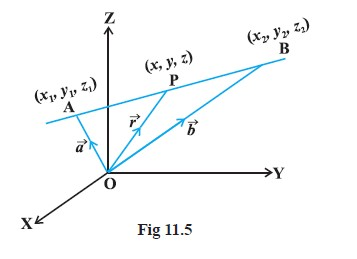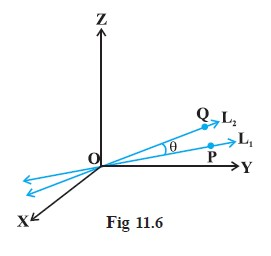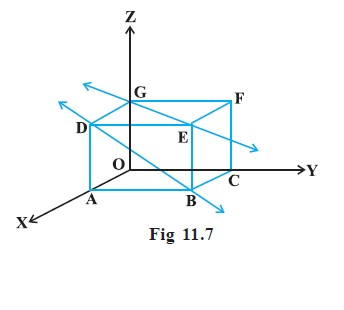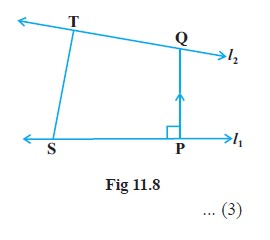Mathematics Equation of a Line in Space, Angle between Two Lines And Shortest Distance between Two Lines

### Topic Covered

color{red} ♦ Equation of a Line in Space
color{red} ♦ Angle between Two Lines
color{red} ♦ Shortest Distance between Two Lines

### Equation of a Line in Space

● We have studied equation of lines in two dimensions, A line is uniquely determined if

(i) it passes through a given point and has given direction, or

(ii) it passes through two given points.

### Equation of a line through a given point and parallel to a given vector vec b● Let vec a be the position vector of the given point

=> Let l be the line which passes through the point A and is parallel to a given vector vec b . Let vec r be the position vector of an arbitrary point P on the line (Fig).

=> Then bar (AP) is parallel to the vector  vec B , I.E.,

BAR (AP)= lambda vec b , where lambda is some real number.

But bar (AP) = bar (OP) - bar (OA)

i.e., color{red}{lambda bar b = vec r - vec a}

Conversely, for each value of the parameter λ, this equation gives the position vector of a point P on the line. Hence, the vector equation of the line is given by

color{green}{vec r = vec a + lambda vec b} .......(1)

color{blue} "Key Concept :"

=> If vec b = a hat i + b hat j + c hat k , then a,b,c are direction ratios of the line and conversely, if a, b, c are direction ratios of a line, then vec b = a hat i + b hat j + c hat k will be the parallel to the line. Here, b should not be confused with | vec b | .

color{brown} "Derivation of cartesian form from vector form"

=> Let the coordinates of the given point A be (x_1, y_1, z_1) and the direction ratios of the line be a, b, c. Consider the coordinates of any point P be (x, y, z). Then

vec r = xhati + yhatj + zhatk ; vec a = x_1 hat i + y_1 hatj + z_1 hatk

and vec b = a hat i + b hatj + c hatk

=> Substituting these values in (1) and equating the coefficients of hat i , hat j  and hat k, we get

x = x_1 + λ a; y = y_1 + λ b; z = z_1+ λ c .......................(2)

=> These are parametric equations of the line. Eliminating the parameter λ from (2),

we get

color{red}{( x - x_1 )/a = (y - y_1 )/b = (z -z_1 )/c} .....................(3)

=> This is the Cartesian equation of the line.

color{red} "Key Piont :" If l, m, n are the direction cosines of the line, the equation of the line is

(x -x_1)/l = (y - y_1)/m = (z -z_1 )/n

Q 3177578486Find the vector and the Cartesian equations of the line through the point
(5, 2, – 4) and which is parallel to the vector 3 hat i + 2 hat j − 8 hat k .
Class 12 Chapter 11 Example 6Solution:

We have

vec a = 5 hat i +2 hat j -4 hat k and  vec b = 3 hat i +2 hat j - 8 hat k

Therefore, the vector equation of the line is

vec r = 5 hat i + 2 hat j - 4 hat k + lambda ( 3 hat i +2 hat j - 8 hat k )

Now, vec r is the position vector of any point P(x, y, z) on the line.

Therefore,  x hat i + y hat j + z hat k = 5 hat i +2 hat j -4 hat k + lambda ( 3 hat i +2 hat j - 8 hat k )

= (5+ 3 lambda) hat i + (2+2 2 lambda) hat j + (-4 -8 lambda) hat k

Eliminating λ , we get

(x-5)/3 = (y-2)/2 = (z+4)/(-8)
which is the equation of the line in Cartesian form.

### Equation of a line passing through two given points=> Let vec a and vec b be the position vectors of two points A (x_1, y_1, z_1) and B(x_2, y_2, z_2), respectively that are lying on a line (Fig 11.5).

=> Let  vec r be the position vector of an arbitrary point P(x, y, z), then P is a point on the line if and only if bar (AP) = vec r - vec a and bar (AB) = vec b - vec a are collinear vectors. Therefore, P is on the line if and only if

vec r - vec a= lambda (vec b - vec a)

or color{blue}{vec r = vec a + lambda (vec b - vec a) , lambda ϵ R} ....(1)

=> This is the vector equation of the line.

color{brown} "Derivation of cartesian form from vector form"

vec r = x hat i + y hat j + z hat k , vec a = x_1 hat i + y_1 hat j + z_1 hat k and vec b = x_2 hat i + y_2 hat j + z_2 hat k ,

Substituting these values in (1), we get

x hat i + y hat j + z hat k = x_1 hat i+ y_1 hat j + z_1 hat k +lambda [ (x_2-x_1) hat i + (y_2-y_1) hat j + (z_2 -z_1) hat k ]

Equating the like coefficients of hat i, hat j, hat k , we get

x =x_1+ lambda (x_2-x_1) ; y = y_1 + lambda (y_2-y_1) ;z = z_1 + lambda (z_2 - z_1)

On eliminating λ, we obtain

 "Equation of line " color{red} {(x -x_1)/( x_2 - x_1) = (y-y_1)/(y_2 - y_1) = (z-z_1)/(z_2- z_1)}

Q 3107578488Find the vector equation for the line passing through the points (–1, 0, 2)
and (3, 4, 6).
Class 12 Chapter 11 Example 7Solution:

Let vec a  and  vec b be the position vectors of the point A(– 1, 0, 2) and B(3, 4, 6).

Then vec a = - hat i + 2 hat k

and vec b = 3 hat i +4 hat j + 6 hat k

Therefore vec b - vec a= 4 hat i +4 hat j + 4 hat k

Let vec r be the position vector of any point on the line. Then the vector equation of
the line is

vec r = - hat i + 2 hat k + lambda (4 hat i + 4 hat j +4 hat k )
Q 3117578489The Cartesian equation of a line is

(x+3)/2 = (y-5)/4 = (z+6)/2

Find the vector equation for the line.
Class 12 Chapter 11 Example 8Solution:

Comparing the given equation with the standard form

(x-x_1)/a = (y-y_1)/b = (z-z_1)/c

We observe that x_1 = -3, y_1 = 5, z_1 = -6 ; a= 2 , b =4, c=2

Thus, the required line passes through the point (– 3, 5, – 6) and is parallel to the
vector 2 hat i + 4 hat j + 2 hat k . Let vec r be the position vector of any point on the line, then the
vector equation of the line is given by

vec r = (-3 hat i + 5 hat j - 6 hat k ) + lambda (2 hat i + 4 hat j +2 hat k)

### Angle between Two Lines=> Let L_1 and L_2 be two lines passing through the origin and with direction ratios a_1, b_1, c_1 and a_2, b_2, c_2, respectively.

=> Let P be a point on L_1 and Q be a point on L_2. Consider the directed lines OP and OQ as given in Fig .

=> Let θ be the acute angle between OP and OQ. Now recall that the directed line segments OP and OQ are vectors with components a_1, b_1, c_1 and a_2, b_2, c_2, respectively. Therefore, the angle θ between them is given by

color{red}{cos theta= | (a_1 a_2 + b_1 b_2 + c_1 c_2 )/(sqrt (a_(1)^2 + b_(1)^2 + c_(1)^2) sqrt ( a_(2)^2 + b_(2)^2 + c_(2)^2) ) |}

=> The angle between the lines in terms of sin θ is given by

sin theta = sqrt(1- cos^2 theta)

= sqrt (1- ( (a_1 a_2 + b_1 b_2 + c_1 c_2 )^2)/( ( a_(1)^2 + b_(1)^2 + c_(1)^2) ( a_(2)^2 + b_(2)^2 + c_(2)^2) ) )

= ( sqrt ( ( a_(1)^2 + b_(1)^2 + c_(1)^2) ( a_(2)^2 + b_(2)^2 + c_(2)^2) - (a_(1) a_2 + b_1 b_2 + c_1 c_2)^2 ) )/(sqrt ( (a_(1)^2 + b_(1)^2 + c_(1)^2) ) sqrt (a_(2)^2 + b_(2)^2 + c_(2)^2) )

color{red}{sintheta= ( sqrt ( (a_1 b_2 - a_2 b_1)^2 + ( b_1 c_2 - b_2 c_1)^2 + ( c_1 a_2 - c_2 a_1)^2 ) )/( sqrt (a_(1)^2 + b_(1)^2 + c_(1)^2) sqrt ( a_(2)^2 + b_(2)^2 + c_(2)^2) )} ................(2)

color{red} "Key Point" - In case the lines L_1 and L_2 do not pass through the origin, we may take lines L'_(1) and L′_(2) which are parallel to L_1 and L_2 respectively and pass through the origin.

\color{green} ✍️ If instead of direction ratios for the lines L_1 and L_2, direction cosines, namely, l_1, m_1, n_1 for L_1 and l_2, m_2, n_2 for L_2 are given, then (1) and (2) takes the following form:

color{red}{cos theta = | l_1 l_2 + m_1 m_2 + n_1 n_2 |}

(as l_(1)^2 + m_(1)^2 + n_(1)^2 =1 = l_(2)^2 + m_(2)^2 + n_(2)^2) .....(3)

and sin theta = sqrt ( (l_1 m_2 - l_2 m_1 )^2 - (m_1 n_2 - m_2 n_1)^2 + (n_1 l_2 - n_2 l_1)^2 ) ....(4)

=> Two lines with direction ratios a_1, b_1, c_1 and a_2, b_2, c_2 are

(i) perpendicular i.e. if θ = 90° by (1)

color{blue}{a_1 a_2 + b_1 b_2 + c_1 c_2 = 0}

(ii) parallel i.e. if θ = 0 by (2)

color{blue}{a_1/a_2 =b_1/b_2 =c_1/c_2}

\color{green} ✍️ Now, we find the angle between two lines when their equations are given. If θ is acute the angle between the lines

vec r = bar (a_1) + lambda bar (b_1) and vec r = bar (a_2) + mu bar ( b_2)

then cos theta= | ( bar (b_1) * bar (b_2) )/( | bar (b_1) | | bar (b_2) | ) |

\color{green} ✍️ So In Cartesian form, if θ is the angle between the lines

 (x-x_1)/(a_1) = (y-y_1)/(b_1) = (z-z_1)/(c_1) ........(1)

and (x-x_2)/(a_2) = (y-y_2)/(b_2) = (z-z_2)/(c_2) ........(2)

where, a_1, b_1, c_1 and a_2, b_2, c_2 are the direction ratios of the lines (1) and (2), respectively, then

\ \ \ \ \ \ \ \ \ \ \ \ \ \ \ \color{red} {cos theta = | (a_1 a_2 + b_1 b_2 + c_1 c_2)/( sqrt (a_(1)^2 + b_(1)^2 + c_(1)^2) sqrt (a_(2)^2 + b_(2)^2 + c_(2)^2) ) |}

Q 3127678581Find the angle between the pair of lines given by

vec r = 3 hat i + 2 hat j - 4 hat k + lambda (hat i +2 hat j + 2 hat k )

and vec r = 5 hat i -2 hat j + mu (3 hat i +2 hat j + 6 hat k)
Class 12 Chapter 11 Example 9Solution:

Here vec (b_1) = hat i + 2 hat j + 2 hat k and vec (b_2) = 3 hat i + 2 hat j + 6 hat k

The angle θ between the two lines is given by

cos theta = | (vec (b_1) * vec ( b_2) )/( | vec b_1 | | vec b_2 | ) | = | ( (hat i +2 hat j +2 hat k)* (3 hat i + 2 hat j + 6 hat k) )/( sqrt (1+4+) sqrt (9+4+36) ) |

= | (3+4+12)/(3 xx 7 ) | = 19/21

Hence theta= cos^(-1) (19/21)
Q 3147678583Find the angle between the pair of lines

(x+3)/3 = (y-1)/5 = (z+3)/4

and (x+1)/1 = ( y-4)/1 = (z-5)/2
Class 12 Chapter 11 Example 10Solution:

The direction ratios of the first line are 3, 5, 4 and the direction ratios of the
second line are 1, 1, 2. If θ is the angle between them, then

cos theta = | (3 * 1 + 5* 1 + 4 *2)/( sqrt (362 + 5^2 + 4^2 ) sqrt (1^2 + 1^2 + 2^2) ) | = 16/(sqrt (50) sqrt 6 ) =16/(5 sqrt 2 sqrt 6) = (8 sqrt 3)/15

Hence, the required angle is cos^(-1) ( (8 sqrt 3)/15)

### Shortest Distance between Two Lines● If two lines in space intersect at a point, then the shortest distance between them is zero.

● if two lines in space are parallel, then the shortest distance between them will be the perpendicular distance, i.e. the length of the perpendicular drawn from a point on one line onto the other line.

● Further, in a space, there are lines which are neither intersecting nor parallel. In fact, such pair of lines are non coplanar and are called skew lines.

=> For example, let us consider a room of size 1, 3, 2 units along x, y and z-axes respectively Fig.

=> The line GE that goes diagonally across the ceiling and the line DB passes through one corner of the ceiling directly above A and goes diagonally down the wall. These lines are skew because they are not parallel and also never meet.● For skew lines, the line of the shortest distance will be perpendicular to both the lines.

### Distance between two skew lines=> We now determine the shortest distance between two skew lines in the following way:

=> Let l_1 and l_2 be two skew lines with equations (Fig.)

vec r = vec (a_1) + lambda vec ( b_1) .........(1)

and vec r= vec (a_2) + mu vec (b_2) .........(2)

=> Take any point S on l_1 with position vector vec (a_1) and T on l_2 , , with position vector vec (a_2)

=> Then the magnitude of the shortest distance vector will be equal to that of the projection of ST along the direction of the line of shortest distance.

=> If bar (PQ) is the shortest distance vector between l_1 and l_2 , then it being perpendicular to both bar ( b_1) and bar(b_2) , the unit vector hat n along bar (PQ) would therefore be

color{orange}{hat n = (vec b_1 xx vec b_2 )/( | vec b_1 xx vec b_2 | )} ...........(3)

Then bar (PQ) = d hat n

=> where, d is the magnitude of the shortest distance vector. Let θ be the angle between bar (ST) and bar (PQ) . Then

PQ=ST | cos theta |

But cos theta = | ( bar (PQ) *bar (ST) )/( | bar(PQ) | | bar (ST) |) |

= | (d hat n * (vec (a_2) - vec (a_1) ) )/( d ST) | ( since bar(ST = vec a_2 - vec a_1 )

= | ( (vec b_1 xx vec b_2 )*( vec a_2 - vec a_1) )/(ST | vec b_1 xx vec b_2 ) | [From (3)]

\color{green} ✍️Hence, the required shortest distance is

d= PQ =ST |cos theta |

or color{blue}{d = | ( (vec b_1 xx vec b_2)* (vec a_2 - vec a_1) )/(| vec b_1 xx vec b_2 |) |}

color{brown} "Shortest Distance In Cartesian form"

The shortest distance between the lines

l_1 : (x-x_1)/(a_1) = (y-y_1)/(b_1) = (z-z_1)/(c_1)

and l_2 : (x-x_2)/(a_2) = (y-y_2)/(b_2) = (z-z_2)/(c_2) is

 \ \ \ \ \"d" = color{red} {( | ( x_2- x_1 , y_2-y_1 , z_2 -z_1), ( a_1 , b_1 , c_1), ( a_2, b_2 , c_2) | )/( sqrt ( (b_1 c_2 - b_2 c_1)^2 + ( c_1 a_2 -c_2 a_1)^2 +(a_1 b_2 - a_2 b_1)^2) )}

### Distance between parallel lines=> If two lines l_1 and l_2 are parallel, then they are coplanar. Let the lines be given by

vec r = vec a_1 + λ vec b ... (1)

and vec r = vec a_2 + μ vec b … (2)

=> where, vec a_1 is the position vector of a point S on l_1 and vec a_2 is the position vector of a point T on l_2 Fig.

=> As l_1, l_2 are coplanar, if the foot of the perpendicular from T on the line l_1 is P, then the distance between the lines l_1 and l_2 = |TP|

=> Let θ be the angle between the vectors bar (ST) and vec b

Then, vec b xx bar (ST) = ( | vec b | | bar (ST) | sin theta) hat n ........(3)

=> where hat n is the unit vector perpendicular to the plane of the lines l_1 and l_2.

But bar (ST ) = vec a_2 - vec a_1

Therefore, from (3), we get

vec b xx (vec a_2 - vec a_1) = | bar b| PT hat n (since PT = ST sin θ)

i.e., | bar b xx ( vec a_2 - vec a_1 ) | = | vec b | PT * 1 (as | hat n | = 1 |

Hence, the distance between the given parallel lines is

 \ \ \ \ \ \ \ \ \ \ \ \ \ \ \color{red} {d= | bar (PT) | = | (vec b xx ( vec a_2 - vec a_1 ) ) /( | vec b | ) |}

Q 3167678585Find the shortest distance between the lines l_1 and l_2 whose vector
equations are

vec r = hat i + hat j + lambda (2 hat i - hat j + hat k) .......(1)

and vec r = 2 hat i + hat j - hat k + mu (3 hat i -5 hat j + 2 hat k) ..(2)

Class 12 Chapter 11 Example 11Solution:

Comparing (1) and (2) with vec = vec a_1 + λ vec b_1
and vec r = vec a_1 + mu vec b_2 respectively

we get  vec a_1 = hat i + hat j , vec b_1 = 2hat i - hat j + hat k

vec a_1 = 2 hat i +hat j - hat k and vec b_2= 3 hat i - 5 hat j + 2 hat k

Therefore vec a_2 - vec a_2 = hat i - hat k

and vec b_1 xx vec b_2 = (2 hat i - hat j + hat k) xx (3 hat i - 5 hat j + 2 hat k)

= | ( hat i , hat j , hat k ), ( 2,-1,1 ), (3,-5,2) | = 3 hat i - hat j - 7 hat k

So | vec b_1 xx vec b_2 | = sqrt(9+1+49) = sqrt (59)

Hence, the shortest distance between the given lines is given by

d= | ( (vec b_1 xx vec b_2) * ( vec a_2 - vec a_1) )/( | vec b_1 xx vec b_2) | = ( | 3- 0 + 7 | )/(sqrt 59) =10/(sqrt 59)
Q 3187678587Find the distance between the lines l_1 and l_2 given by

vec r = hat i + 2 hat j -4 hat k + lambda (2 hat i +3 hat j + 6 hat k)

and vec r = 3 hat i + 3 hat j - 5 hat k + mu (2 hat i +3 hat j +6 hat k )
Class 12 Chapter 11 Example 12Solution:

The two lines are parallel (Why? ) We have

vec a_1 = ht i + 2 hat j -4 hat k , vec a_2 = 3 hat i + 3 hat j - 5 hat k and vec b = 2 hat i + 3 hat j + 6 hat k

Therefore, the distance between the lines is given by

d = | (vec b xx (vec a_2 - vec a_1) )/( sqrt 49) | = (sqrt 293)/( sqrt 49) = (sqrt 293)/7